# Multiplexing Introduction R Multiplexing R The set of

• Slides: 25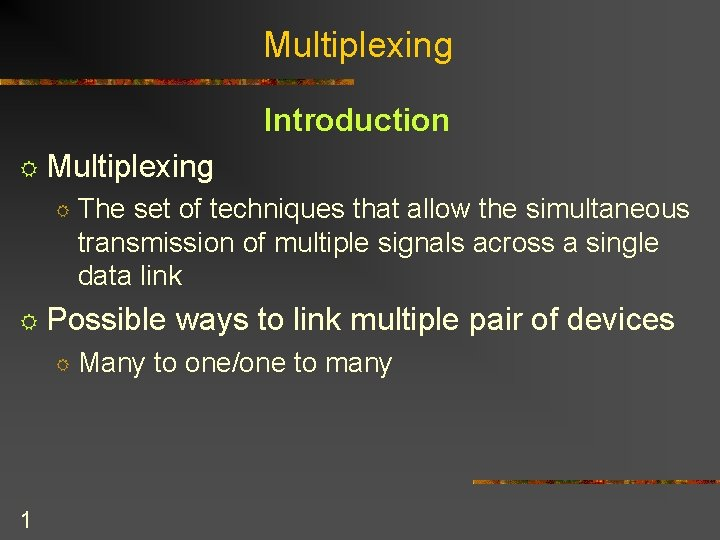Multiplexing Introduction R Multiplexing R The set of techniques that allow the simultaneous transmission of multiple signals across a single data link R Possible R 1 ways to link multiple pair of devices Many to one/one to many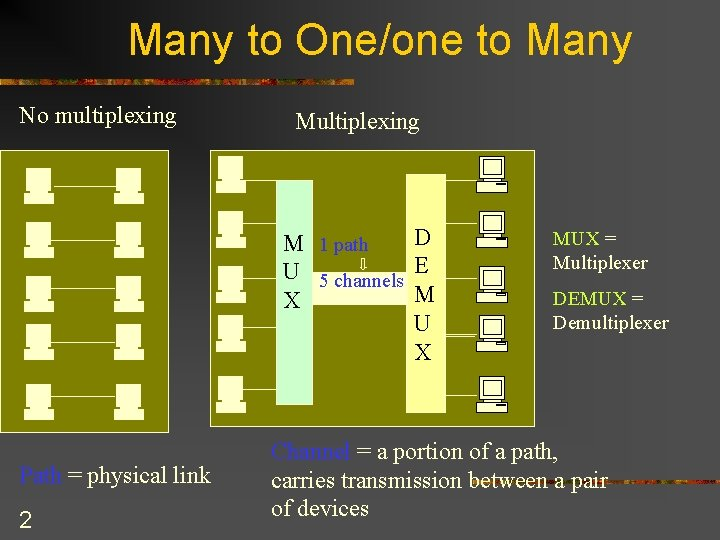Many to One/one to Many No multiplexing Multiplexing D M 1 path U 5 channels E M X U X Path = physical link 2 MUX = Multiplexer DEMUX = Demultiplexer Channel = a portion of a path, carries transmission between a pair of devices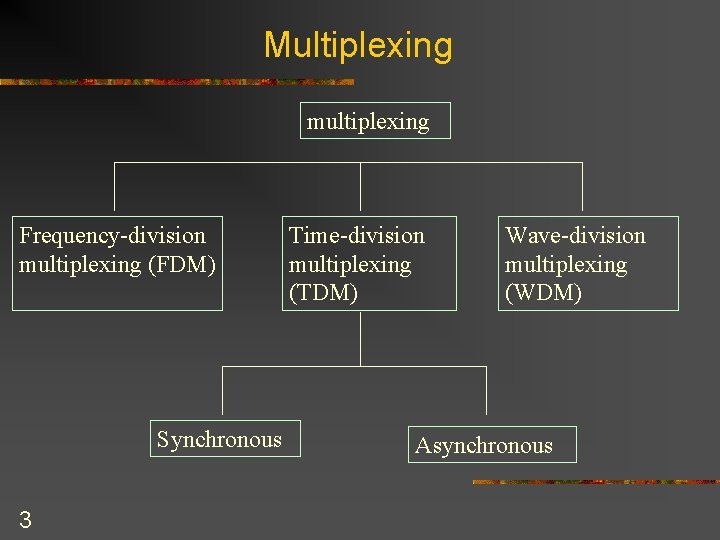Multiplexing multiplexing Frequency-division multiplexing (FDM) Synchronous 3 Time-division multiplexing (TDM) Wave-division multiplexing (WDM) AsynchronousFrequency-Division Multiplexing FDM Process Guard band M Channel 1 U Channel 2 X Channel 3 D E M U X Analog technique Link bandwidth > sum (channel bandwidth) Similar to road traffic merging into one 4 multilane-roadFDM Process R Process A sending device generates signal R A multiplexer R modulates each signal into different frequencies (f 1, f 2, f 3, etc. ) R Combines them into a composite signal R Sends it over a media link R R A demultilexer R Uses a series of filters to decompose the multiplexed signal individual signals demodulators R Demodulators separate signals from their carriers and pass them to the waiting receivers 5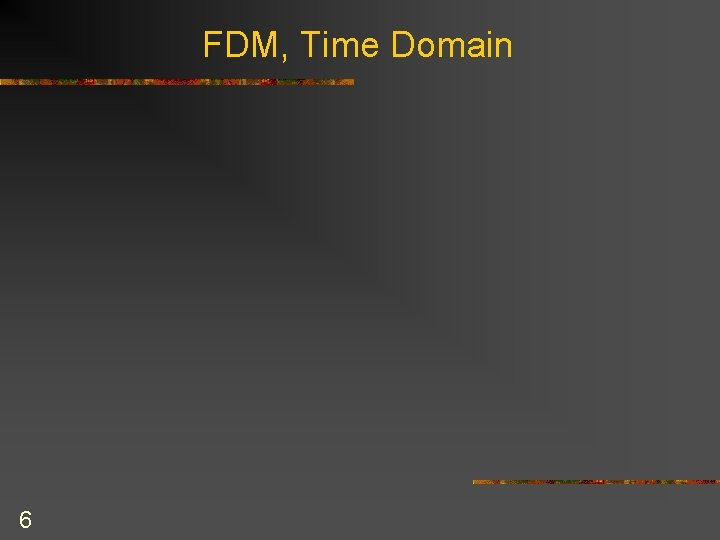FDM, Time Domain 6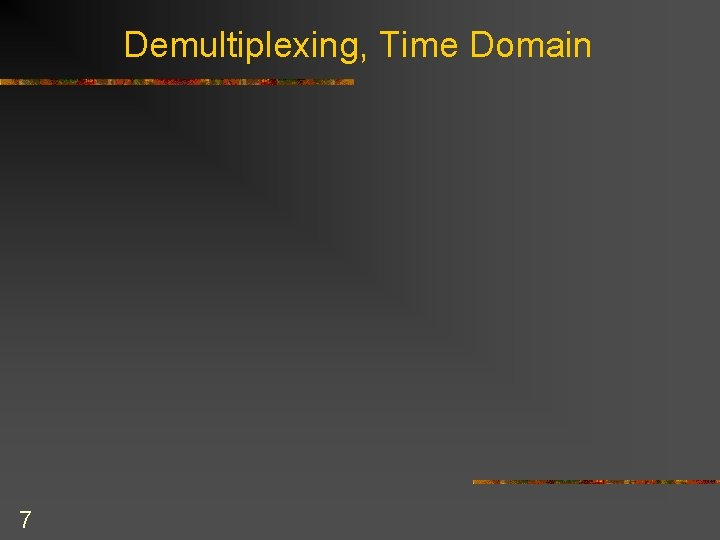Demultiplexing, Time Domain 7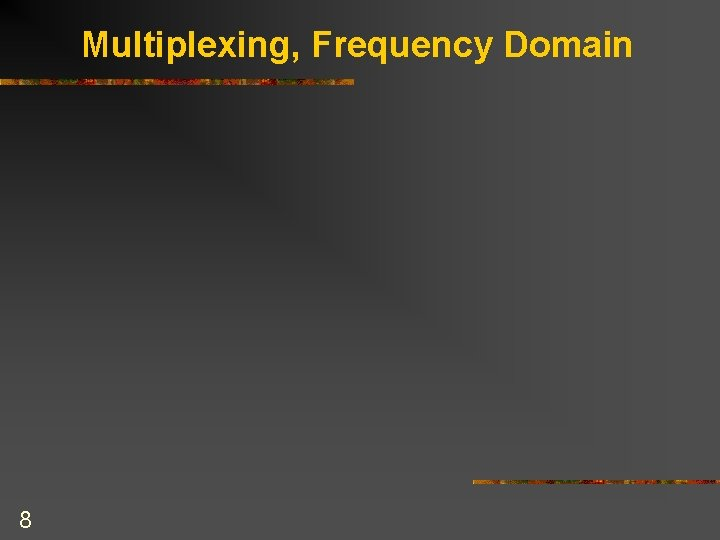Multiplexing, Frequency Domain 8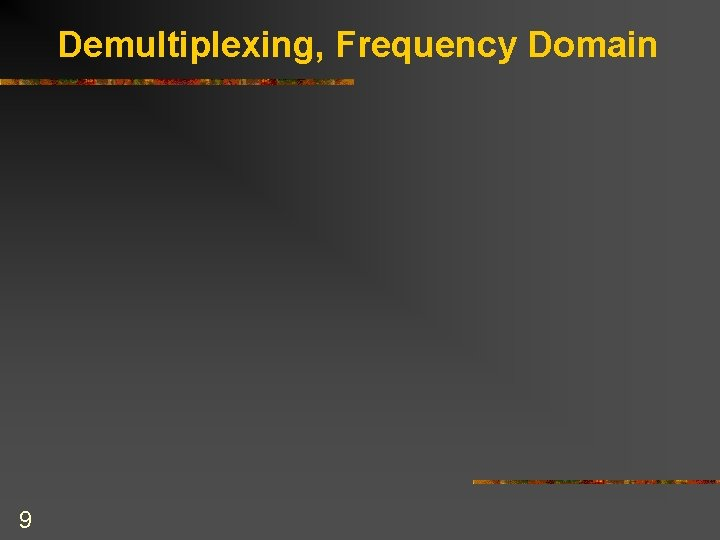Demultiplexing, Frequency Domain 9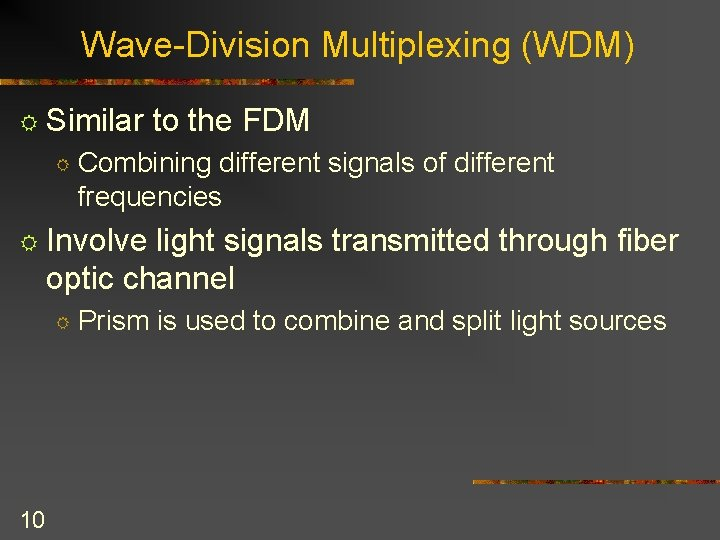Wave-Division Multiplexing (WDM) R Similar R to the FDM Combining different signals of different frequencies R Involve light signals transmitted through fiber optic channel R 10 Prism is used to combine and split light sources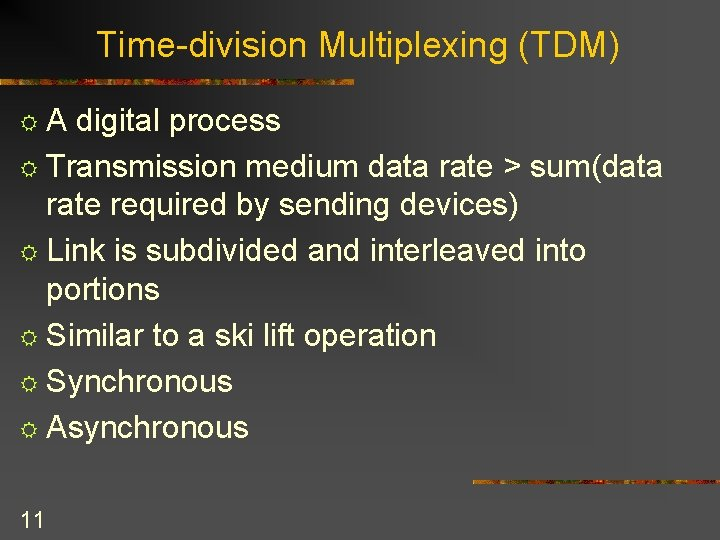Time-division Multiplexing (TDM) RA digital process R Transmission medium data rate > sum(data rate required by sending devices) R Link is subdivided and interleaved into portions R Similar to a ski lift operation R Synchronous R Asynchronous 11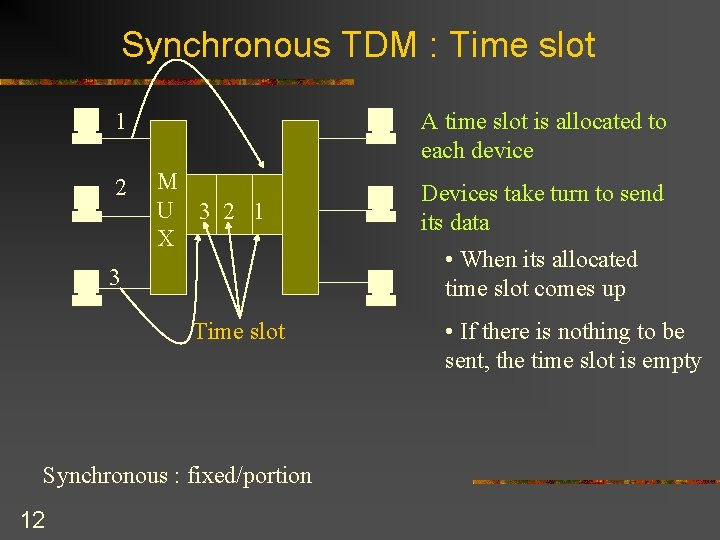Synchronous TDM : Time slot 1 2 M U 3 2 1 X 3 D E M U X Time slot Synchronous : fixed/portion 12 A time slot is allocated to each device Devices take turn to send its data • When its allocated time slot comes up • If there is nothing to be sent, the time slot is emptySynchronous TDM : Frame n M U X Frame 2 Frame 1 …. Number of inputs : 3 Number of slots in each frame : 3 13 One or more slots dedicated to each sending device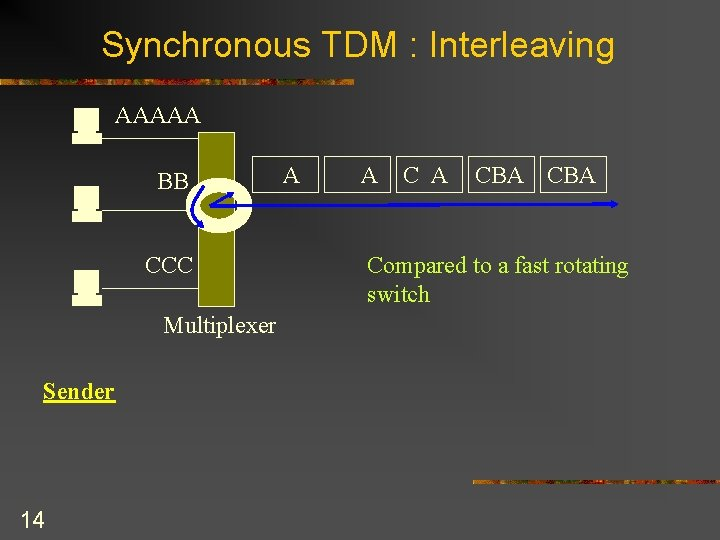Synchronous TDM : Interleaving AAAAA BB CCC Multiplexer Sender 14 A A CBA Compared to a fast rotating switchSynchronous TDM : Interleaving AAAAA A A CBA CBA BB CCC Demultiplexer Weakness : wasted slots 15Synchronous TDM : Framing Bits A 1 A 0 C A 1 CBA 0 10101 Synchronization pattern 16 One or more synchronization bits are added to the beginning of each frame, to help the demultiplexer separate the time slots accurately CBA 1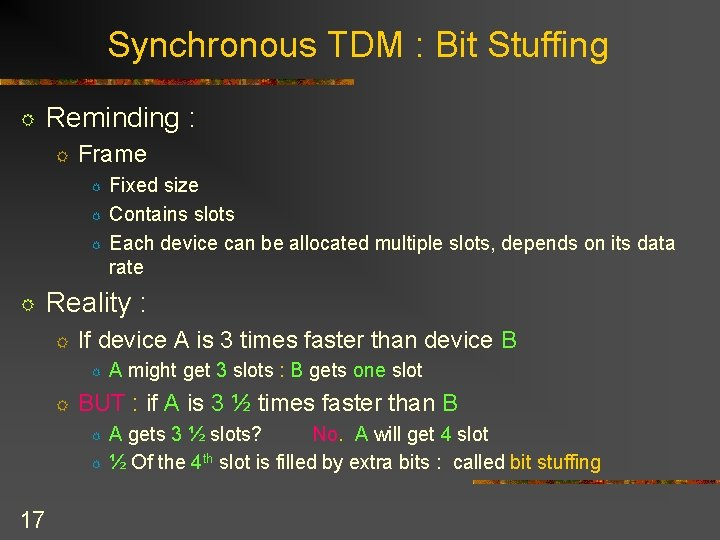Synchronous TDM : Bit Stuffing R Reminding : R Frame R R Reality : R If device A is 3 times faster than device B R R A might get 3 slots : B gets one slot BUT : if A is 3 ½ times faster than B R R 17 Fixed size Contains slots Each device can be allocated multiple slots, depends on its data rate A gets 3 ½ slots? No. A will get 4 slot ½ Of the 4 th slot is filled by extra bits : called bit stuffing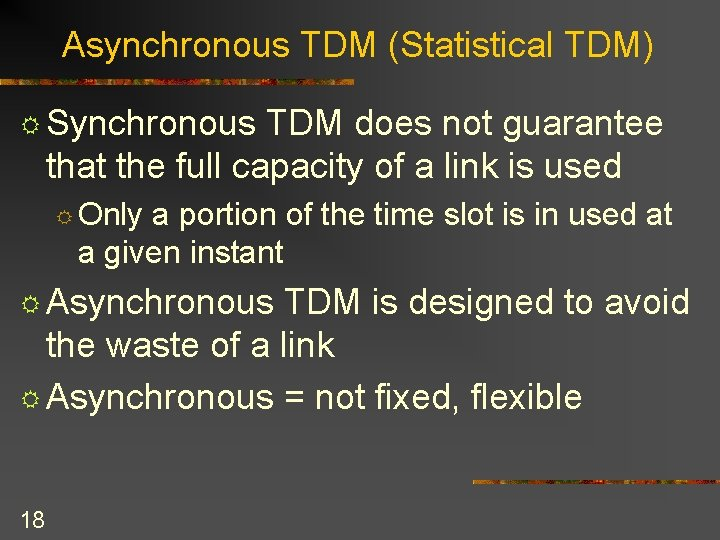Asynchronous TDM (Statistical TDM) R Synchronous TDM does not guarantee that the full capacity of a link is used R Only a portion of the time slot is in used at a given instant R Asynchronous TDM is designed to avoid the waste of a link R Asynchronous = not fixed, flexible 18Asynchronous TDM R Sum (speed of all input lines ) <= link capacity R If there are n input lines R The frame will contain no more than m slots, where m < n R Asynchronous TDM can support more devices than a synchronous TDM 19Asynchronous TDM Frame 2 Frame n M U X …. Number of inputs : 5 Number of slots in each frame : 3 20 Frame 1Asynchronous TDM R The number of time slots in an async TDM is based on a statistical analysis of the number of input lines that are likely to be transmitting at any given time R How it works : the multiplexer Scans the input lines R Accepts portions of data until a frame is filled R Sends the frame across the link R If there are not enough data to fill the frame, R R The frame is transmitted on partially-filled R Transmits the frame 21Example of TDM Frames a. Only three lines sending data 22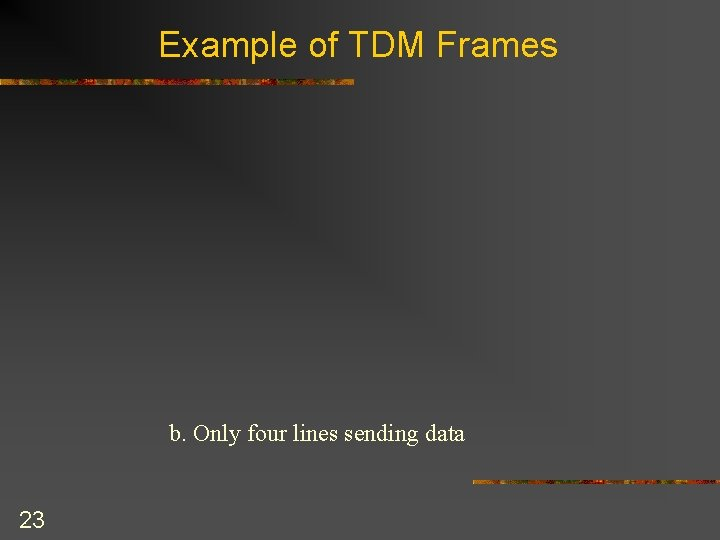Example of TDM Frames b. Only four lines sending data 23Example of TDM Frames c. All five lines sending data 24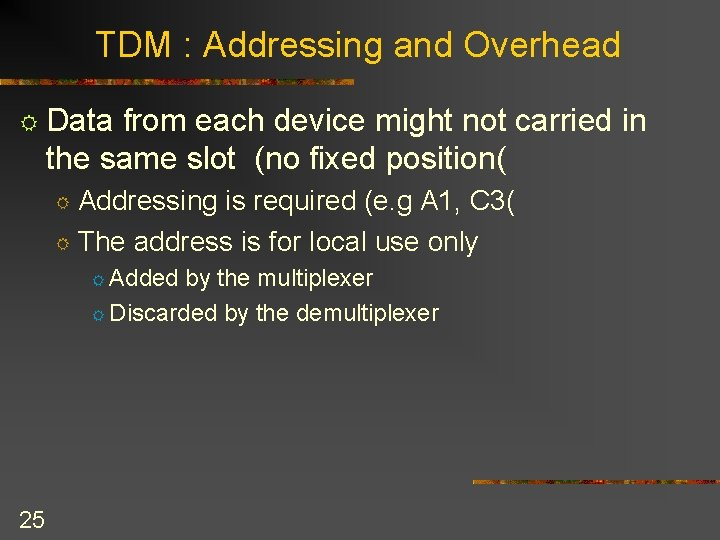TDM : Addressing and Overhead R Data from each device might not carried in the same slot (no fixed position( Addressing is required (e. g A 1, C 3( R The address is for local use only R R Added by the multiplexer R Discarded by the demultiplexer 25